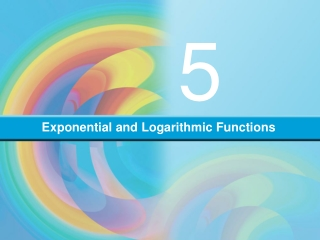DownloadDownload PresentationExponential and Logarithmic Functions

# Exponential and Logarithmic Functions

Télécharger la présentation## Exponential and Logarithmic Functions

- - - - - - - - - - - - - - - - - - - - - - - - - - - E N D - - - - - - - - - - - - - - - - - - - - - - - - - - -
##### Presentation Transcript

1. 5 Exponential and Logarithmic Functions

2. EXPONENTIAL AND LOGARITHMIC FUNCTIONS 5.2 Applications of Exponential Functions Objectives • Solve compound interest problems. • Solve exponential growth and decay problems.

3. Compound Interest Compound interest provides another illustration of exponential growth. Suppose that \$500 (called the principal) is invested at an interest rate of 8% compounded annually. The interest earned the first year is \$500(0.08) = \$40, and this amount is added to the original \$500 to form a new principal of \$540 for the second year. The interest earned during the second year is \$540(0.08) = \$43.20, and this amount is added to \$540 to form a new principal of \$583.20 for the third year. Each year a new principal is formed by reinvesting the interest earned during that year.

4. Compound Interest In general, suppose that a sum of money P (called the principal) is invested at an interest rate of r% compounded annually. The interest earned the first year is Pr, and the new principal for the second year is P + Pr or P(1 + r). Note that the new principal for the second year can be found by multiplying the original principal P by (1 + r). In like fashion, we can find the new principal for the third year by multiplying the previous principal, P(1 + r), by 1 + r, thus obtaining P(1 + r)2. If this process is continued, then after t years the total amount of money accumulated, A, is given by

5. Compound Interest Problem 1 What rate of interest is needed for an investment of \$1000 to yield \$3000 in 10 years if the interest is compounded annually?

6. Compound Interest Problem 1 Solution: Let’s substitute \$1000 for P, \$3000 for A, and 10 years for t in the compound interest formula and solve for r: A = P(1 + r)t 3000 = 1000(1 + r)10 3 = (1 + r)10 30.1 = [(1 + r)10]0.1Raise both sides to the 0.1 power 1.116123174 1 + r 0.116123174 r r = 11.6% to the nearest tenth of a percent Therefore a rate of interest of approximately 11.6% is needed.

7. Compound Interest If money invested at a certain rate of interest is to be compounded more than once a year, then the basic formula A = P(1 + r)tcan be adjusted according to the number of compounding periods in a year. For example, for compounding semiannually, the formula becomes and for compounding quarterly, the formula becomes In general, if n represents the number of compounding periods in a year, then the formula becomes

8. Exponential Decay An example of exponential decay involves radioactive substances. The rate of decay can be described exponentially and is based on the half-life of a substance. The half-life of a radioactive substance is the amount of time that it takes for one-half of an initial amount of the substance to disappear as the result of decay. Suppose there is an initial amount, Q0, of a radioactive substance with a half-life of h. The amount of substance remaining, Q, after a time period of t is given by the formula The units of measure for t and h must be the same.

9. Exponential Decay Problem 2 Barium-140 has a half-life of 13 days. If there are 500 milligrams of barium initially, how many milligrams remain after 26 days? After 100 days?

10. Exponential Decay Problem 2 Solution: With Q0 = 500 and h = 13, the half-life formula becomes If t = 26, then

11. Exponential Decay Problem 2 Thus 125 milligrams remain after 26 days. If t = 100, then to the nearest tenth of a milligram Approximately 2.4 milligrams remain after 100 days.

12. Number e The function defined by the equation f (x) = exis the natural exponential function.

13. Back to Compound Interest Let’s return to the concept of compound interest. If the number of compounding periods in a year is increased indefinitely, we arrive at the concept of compounding continuously. Mathematically, we can do this by applying the limit concept to the expression The formula yields the accumulated value, A, of a sum of money, P, that has been invested for t years at a rate of r% compounded continuously.

14. Law of Exponential Growth The law of exponential growth, is used as a mathematical model for numerous growth-and-decay applications. In this equation, Q(t) represents the quantity of a given substance at any time t; Q0 is the initial amount of the substance (when t = 0), and k is a constant that depends on the particular application. If k < 0, then Q(t) decreases as t increases, and we refer to the model as the law of decay.

15. Law of Exponential Growth Problem 3 Suppose that in a certain culture, the equation Q(t) = 15,000e0.3texpresses the number of bacteria present as a function of the time t, where t is expressed in hours. Find (a) the initial number of bacteria, and (b) the number of bacteria after 3 hours.

16. Law of Exponential Growth Problem 3 Solutions: a. The initial number of bacteria is produced when t = 0. Q(0) = 15,000e0.3(0) = 15,000e0 = 15,000 e0 = 1 b. Q(3) = 15,000e0.3(3) = 15,000e0.9 = 36,894 to the nearest whole number There should be approximately 36,894 bacteria present after 3 hours.